# Tensorflow_cookbook

Code for Tensorflow Machine Learning Cookbook
Alternatives To Tensorflow_cookbook
Tensorflow177,8673277717 hours ago46October 23, 20192,043apache-2.0C++
An Open Source Machine Learning Framework for Everyone
Transformers112,634641,86914 hours ago114July 18, 2023866apache-2.0Python
🤗 Transformers: State-of-the-art Machine Learning for Pytorch, TensorFlow, and JAX.
Keras59,44757815 hours ago80June 27, 202398apache-2.0Python
Deep Learning for humans
Tensorflow Examples42,312
a year ago218otherJupyter Notebook
TensorFlow Tutorial and Examples for Beginners (support TF v1 & v2)
Photoprism28,81142 days ago151April 25, 2021389otherGo
AI-Powered Photos App for the Decentralized Web 🌈💎✨
Ray27,9548029817 hours ago87July 24, 20233,448apache-2.0Python
Ray is a unified framework for scaling AI and Python applications. Ray consists of a core distributed runtime and a set of AI Libraries for accelerating ML workloads.
Data Science Ipython Notebooks25,242
3 months ago34otherPython
Data science Python notebooks: Deep learning (TensorFlow, Theano, Caffe, Keras), scikit-learn, Kaggle, big data (Spark, Hadoop MapReduce, HDFS), matplotlib, pandas, NumPy, SciPy, Python essentials, AWS, and various command lines.
Handson Ml25,030
3 months ago139apache-2.0Jupyter Notebook
⛔️ DEPRECATED – See https://github.com/ageron/handson-ml3 instead.
Handson Ml224,694
5 months ago197apache-2.0Jupyter Notebook
A series of Jupyter notebooks that walk you through the fundamentals of Machine Learning and Deep Learning in Python using Scikit-Learn, Keras and TensorFlow 2.
Netron24,090469a day ago587August 01, 202323mitJavaScript
Visualizer for neural network, deep learning, and machine learning models
Alternatives To Tensorflow_cookbook
Select To Compare

Alternative Project Comparisons# TensorFlow Machine Learning Cookbook

## A Packt Publishing Book

### By Nick McClure

=================

=================

## Ch 1: Getting Started with TensorFlow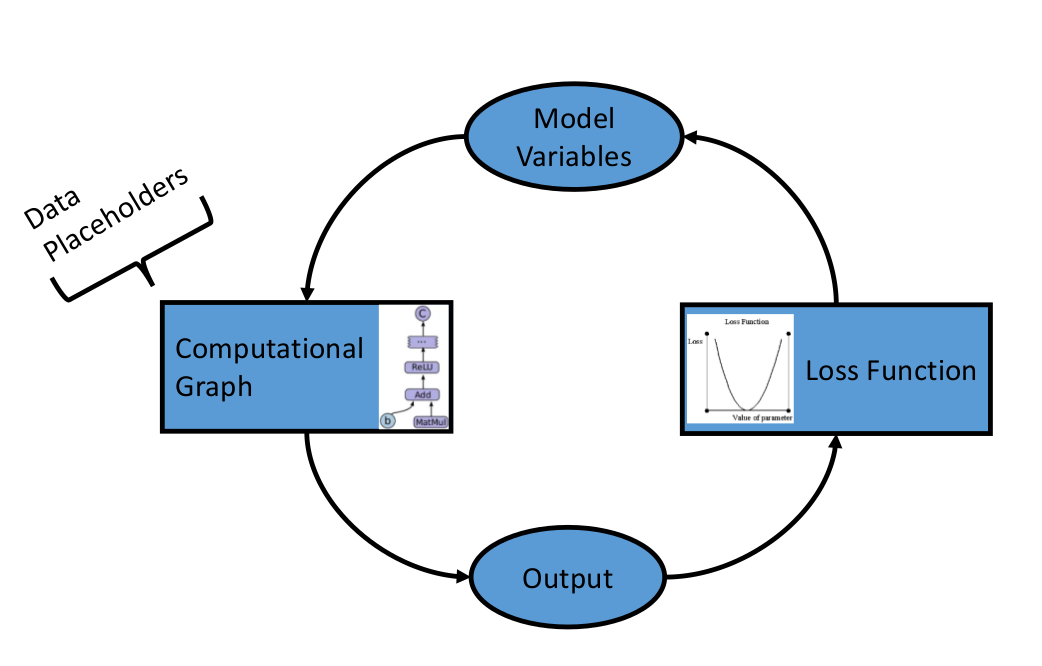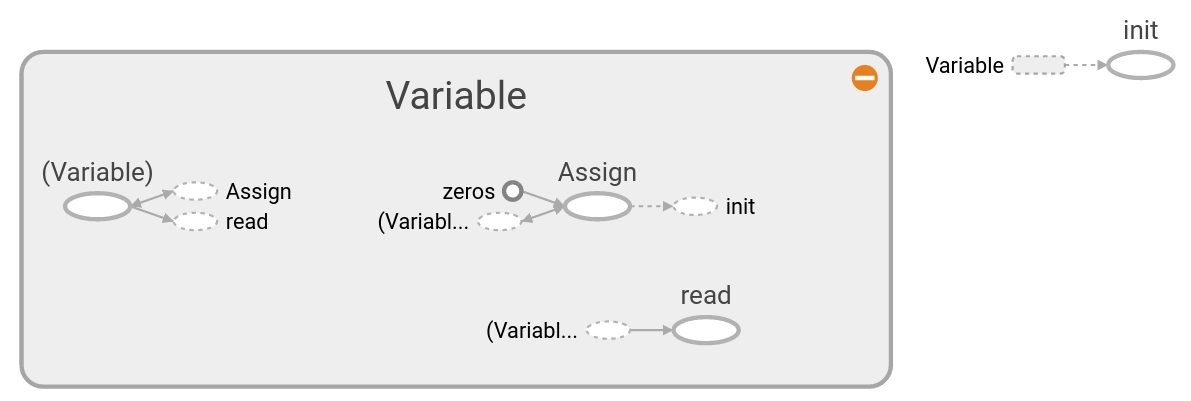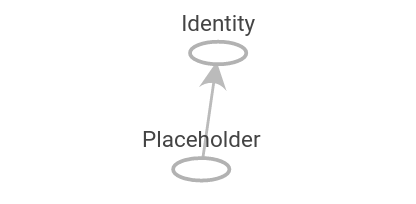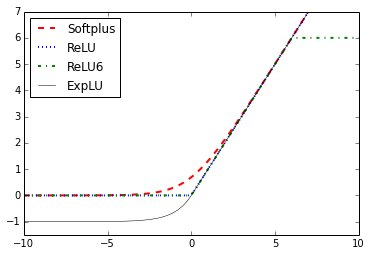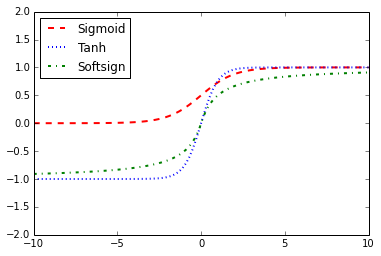This chapter intends to introduce the main objects and concepts in TensorFlow. We also introduce how to access the data for the rest of the book and provide additional resources for learning about TensorFlow.

• Here we introduce TensorFlow and the general outline of how most TensorFlow algorithms work.
• How to create and initialize tensors in TensorFlow. We also depict how these operations appear in Tensorboard.
• How to create and use variables and placeholders in TensorFlow. We also depict how these operations appear in Tensorboard.
• Understanding how TensorFlow can work with matrices is crucial to understanding how the algorithms work.
• How to use various mathematical operations in TensorFlow.
• Activation functions are unique functions that TensorFlow has built in for your use in algorithms.
• Here we show how to access all the various required data sources in the book. There are also links describing the data sources and where they come from.
• Mostly official resources and papers. The papers are TensorFlow papers or Deep Learning resources.

## Ch 2: The TensorFlow Way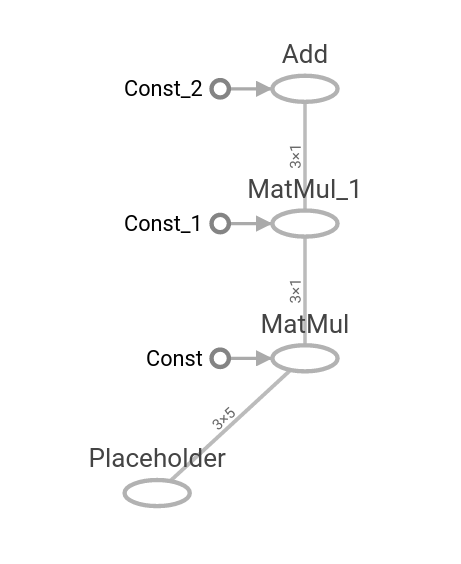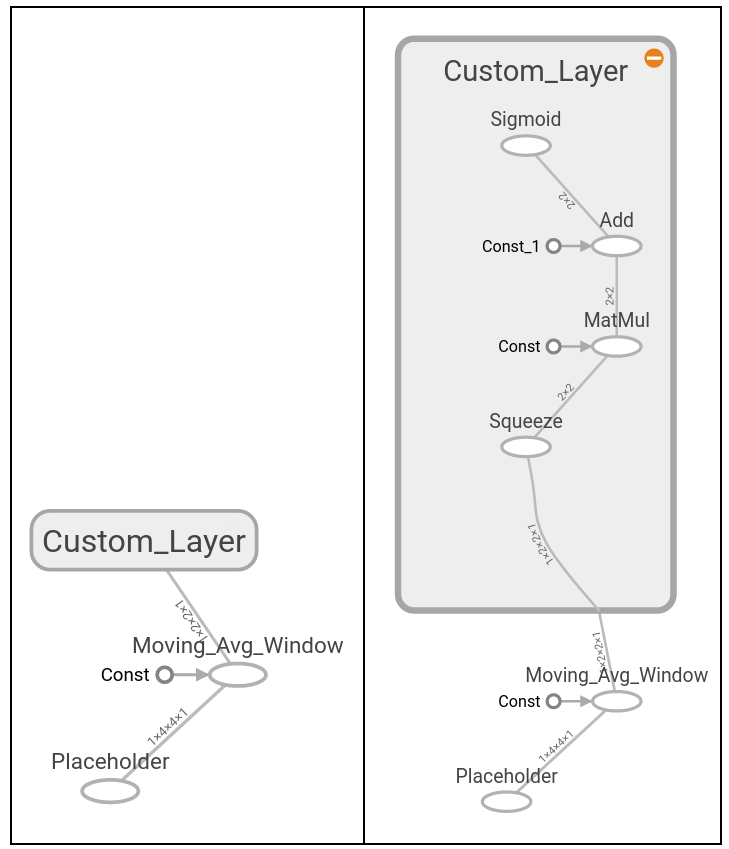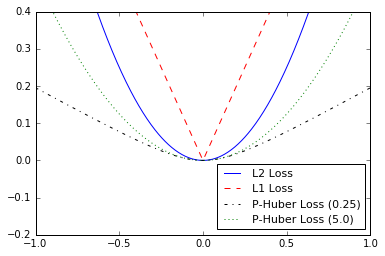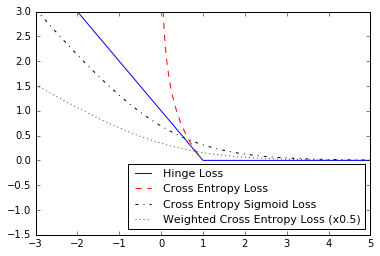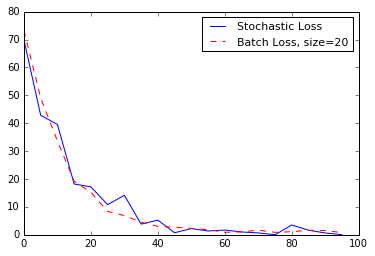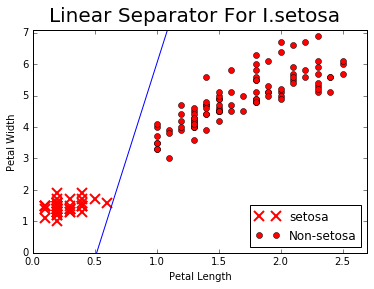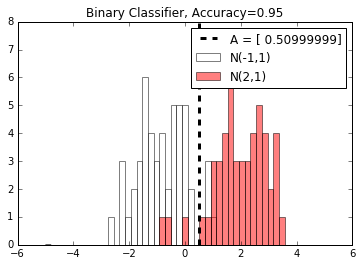After we have established the basic objects and methods in TensorFlow, we now want to establish the components that make up TensorFlow algorithms. We start by introducing computational graphs, and then move to loss functions and back propagation. We end with creating a simple classifier and then show an example of evaluating regression and classification algorithms.

• We show how to create an operation on a computational graph and how to visualize it using Tensorboard.
• We show how to create multiple operations on a computational graph and how to visualize them using Tensorboard.
• Here we extend the usage of the computational graph to create multiple layers and show how they appear in Tensorboard.
• In order to train a model, we must be able to evaluate how well it is doing. This is given by loss functions. We plot various loss functions and talk about the benefits and limitations of some.
• Here we show how to use loss functions to iterate through data and back propagate errors for regression and classification.
• TensorFlow makes it easy to use both batch and stochastic training. We show how to implement both and talk about the benefits and limitations of each.
• We now combine everything together that we have learned and create a simple classifier.
• Any model is only as good as it's evaluation. Here we show two examples of (1) evaluating a regression algorithm and (2) a classification algorithm.

## Ch 3: Linear Regression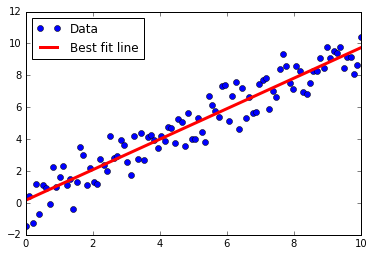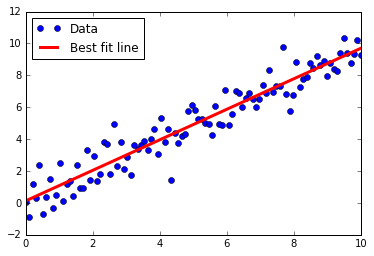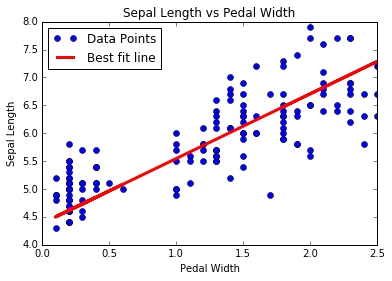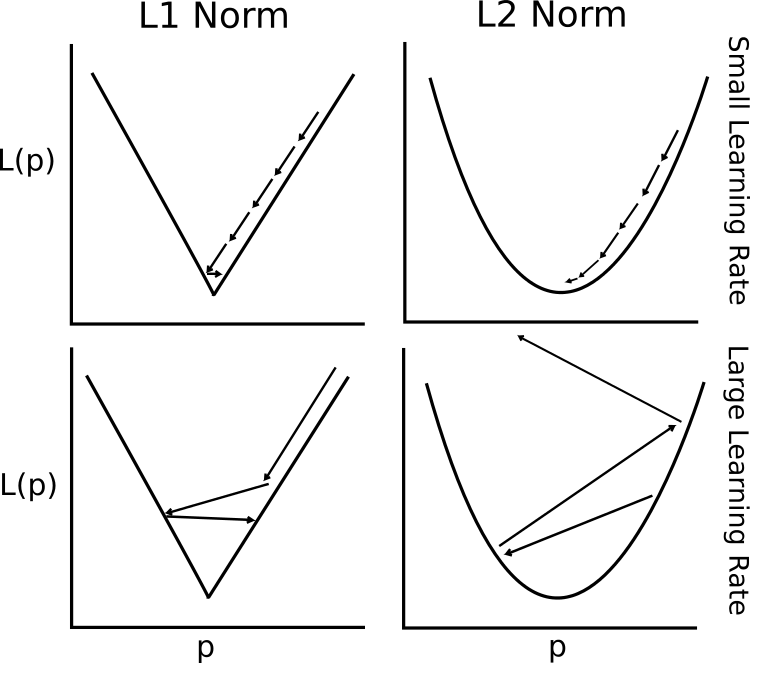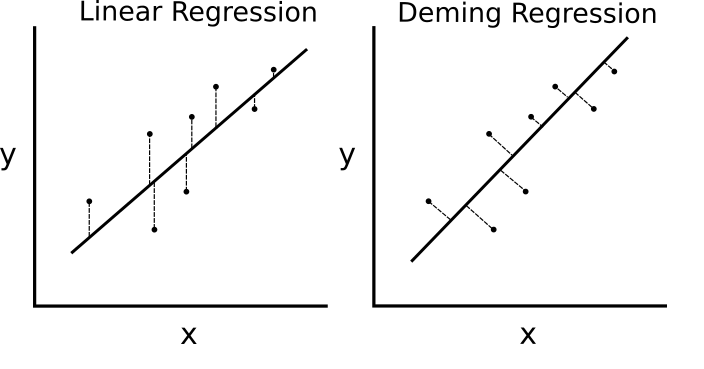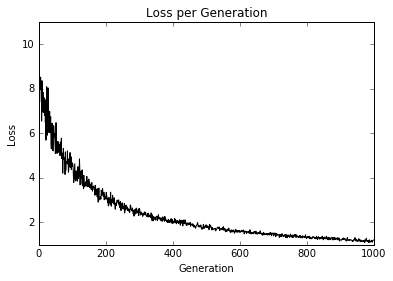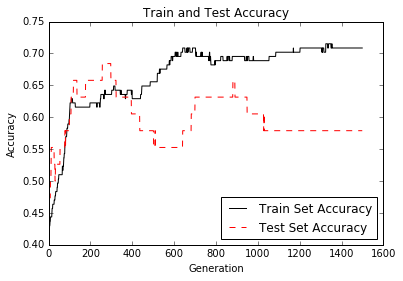Here we show how to implement various linear regression techniques in TensorFlow. The first two sections show how to do standard matrix linear regression solving in TensorFlow. The remaining six sections depict how to implement various types of regression using computational graphs in TensorFlow.

• How to solve a 2D regression with a matrix inverse in TensorFlow.
• Solving a 2D linear regression with Cholesky decomposition.
• Linear regression iterating through a computational graph with L2 Loss.
• L2 vs L1 loss in linear regression. We talk about the benefits and limitations of both.
• Deming (total) regression implemented in TensorFlow by changing the loss function.
• Lasso and Ridge regression are ways of regularizing the coefficients. We implement both of these in TensorFlow via changing the loss functions.
• Elastic net is a regularization technique that combines the L2 and L1 loss for coefficients. We show how to implement this in TensorFlow.
• We implement logistic regression by the use of an activation function in our computational graph.

## Ch 4: Support Vector Machines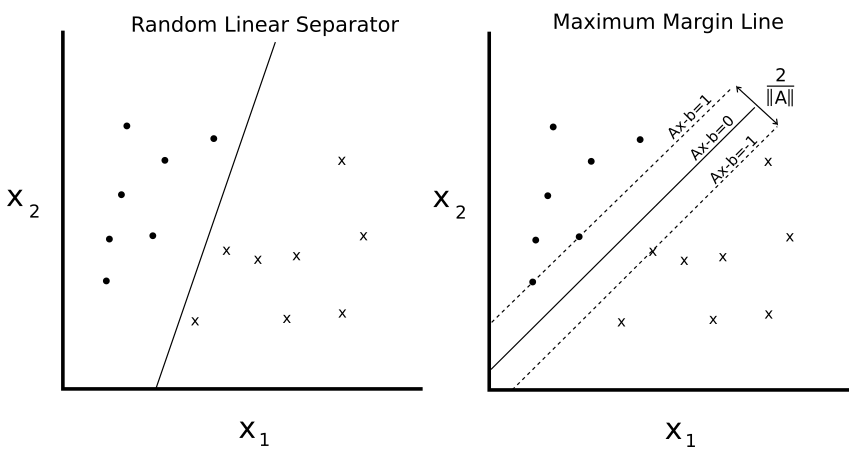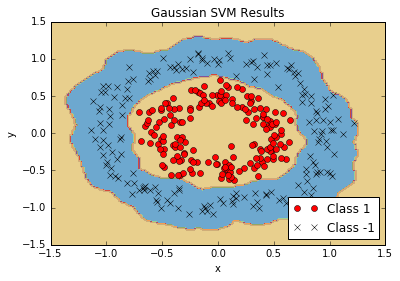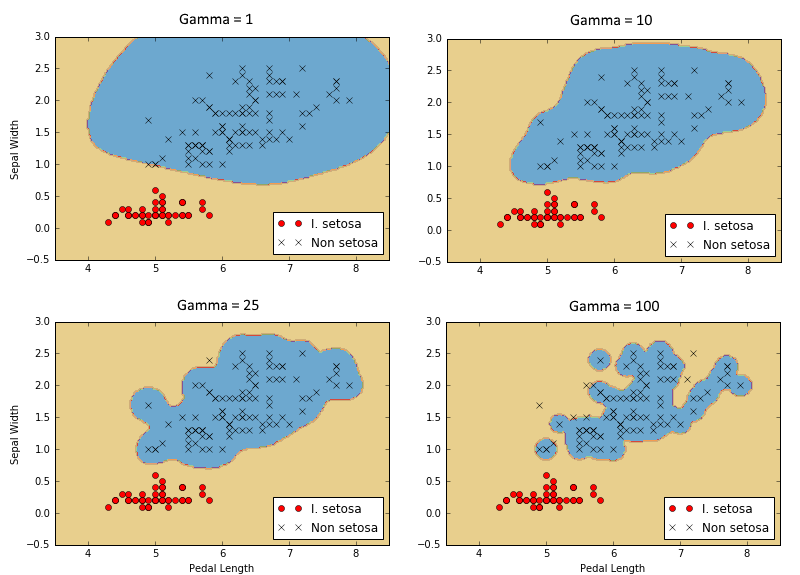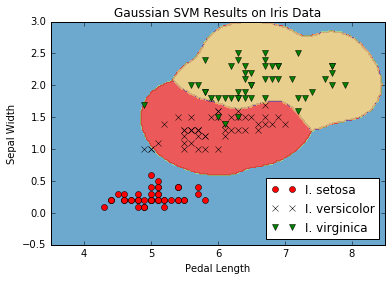This chapter shows how to implement various SVM methods with TensorFlow. We first create a linear SVM and also show how it can be used for regression. We then introduce kernels (RBF Gaussian kernel) and show how to use it to split up non-linear data. We finish with a multi-dimensional implementation of non-linear SVMs to work with multiple classes.

• We introduce the concept of SVMs and how we will go about implementing them in the TensorFlow framework.
• We create a linear SVM to separate I. setosa based on sepal length and pedal width in the Iris data set.
• The heart of SVMs is separating classes with a line. We change tweek the algorithm slightly to perform SVM regression.
• In order to extend SVMs into non-linear data, we explain and show how to implement different kernels in TensorFlow.
• We use the Gaussian kernel (RBF) to separate non-linear classes.
• SVMs are inherently binary predictors. We show how to extend them in a one-vs-all strategy in TensorFlow.

## Ch 5: Nearest Neighbor Methods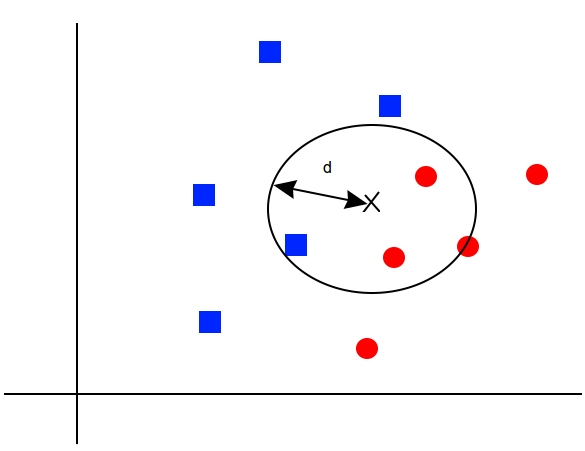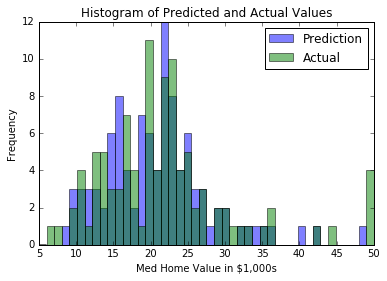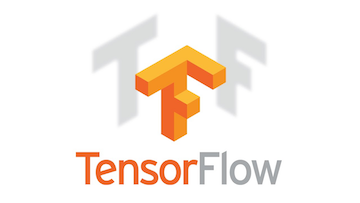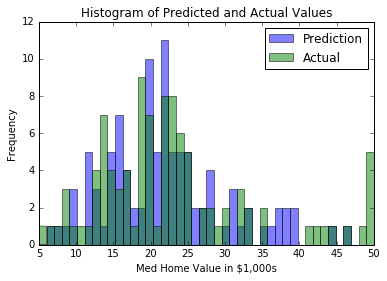Nearest Neighbor methods are a very popular ML algorithm. We show how to implement k-Nearest Neighbors, weighted k-Nearest Neighbors, and k-Nearest Neighbors with mixed distance functions. In this chapter we also show how to use the Levenshtein distance (edit distance) in TensorFlow, and use it to calculate the distance between strings. We end this chapter with showing how to use k-Nearest Neighbors for categorical prediction with the MNIST handwritten digit recognition.

• We introduce the concepts and methods needed for performing k-Nearest Neighbors in TensorFlow.
• We create a nearest neighbor algorithm that tries to predict housing worth (regression).
• In order to use a distance function on text, we show how to use edit distances in TensorFlow.
• Here we implement scaling of the distance function by the standard deviation of the input feature for k-Nearest Neighbors.
• We use a mixed distance function to match addresses. We use numerical distance for zip codes, and string edit distance for street names. The street names are allowed to have typos.
• The MNIST digit image collection is a great data set for illustration of how to perform k-Nearest Neighbors for an image classification task.

## Ch 6: Neural Networks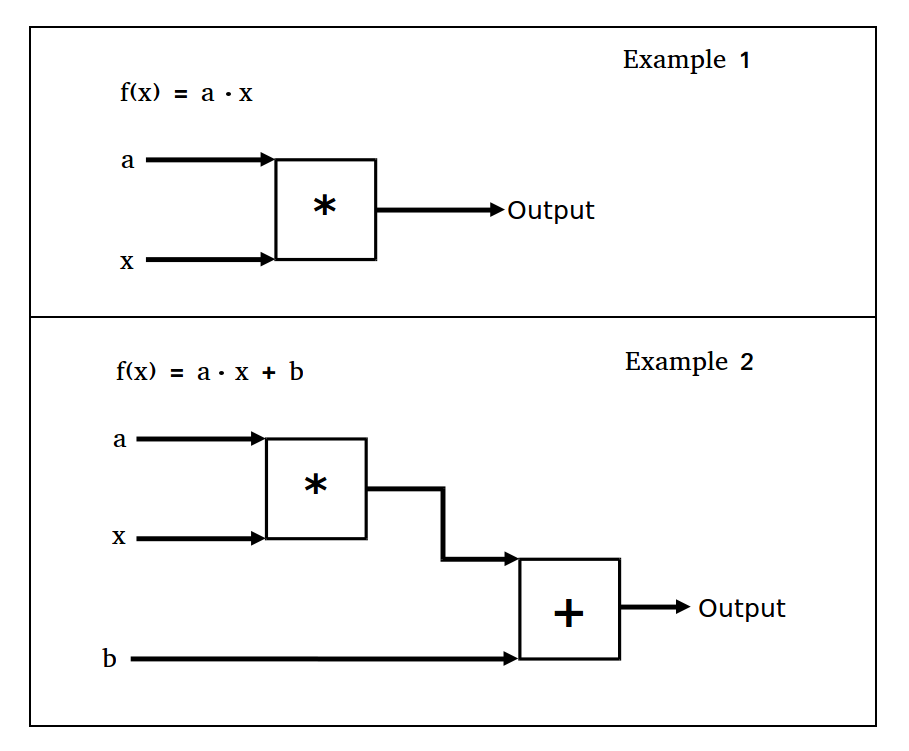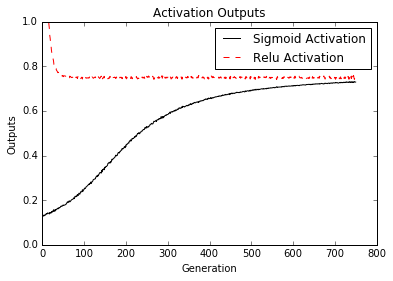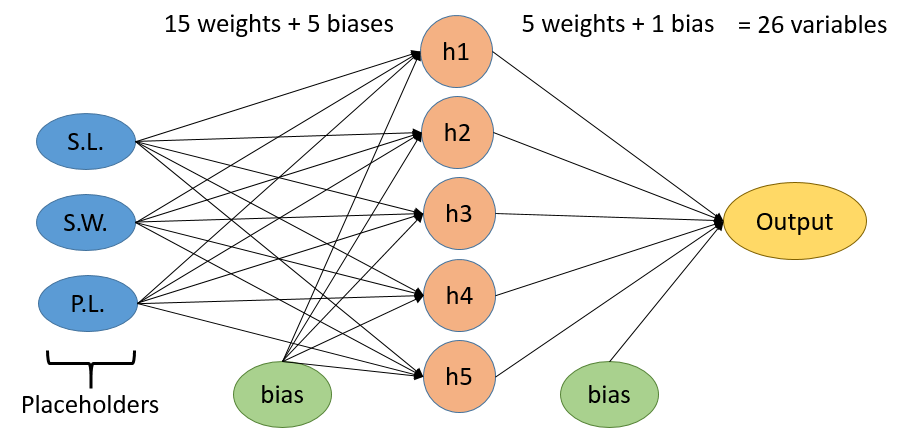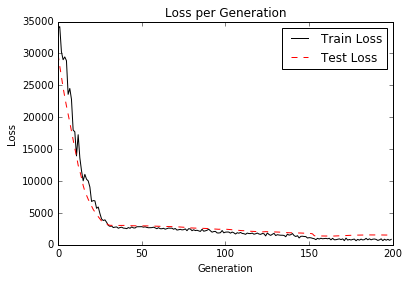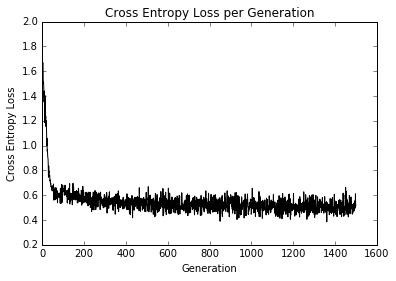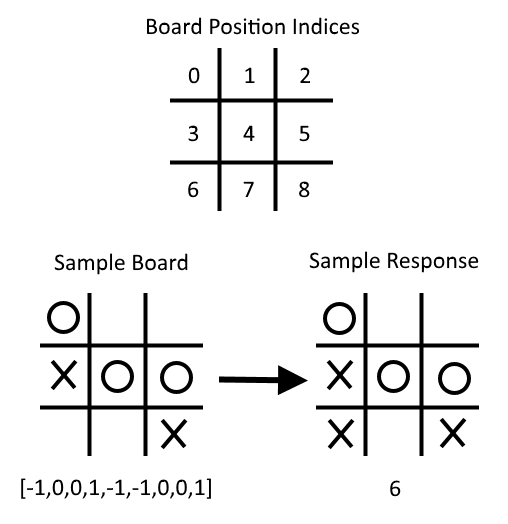Neural Networks are very important in machine learning and growing in popularity due to the major breakthroughs in prior unsolved problems. We must start with introducing 'shallow' neural networks, which are very powerful and can help us improve our prior ML algorithm results. We start by introducing the very basic NN unit, the operational gate. We gradually add more and more to the neural network and end with training a model to play tic-tac-toe.

• We introduce the concept of neural networks and how TensorFlow is built to easily handle these algorithms.
• We implement an operational gate with one operation. Then we show how to extend this to multiple nested operations.
• Now we have to introduce activation functions on the gates. We show how different activation functions operate.
• We have all the pieces to start implementing our first neural network. We do so here with regression on the Iris data set.
• This section introduces the convolution layer and the max-pool layer. We show how to chain these together in a 1D and 2D example with fully connected layers as well.
• Here we show how to functionalize different layers and variables for a cleaner multi-layer neural network.
• We show how we can improve the convergence of our prior logistic regression with a set of hidden layers.
• Given a set of tic-tac-toe boards and corresponding optimal moves, we train a neural network classification model to play. At the end of the script, you can attempt to play against the trained model.

## Ch 7: Natural Language Processing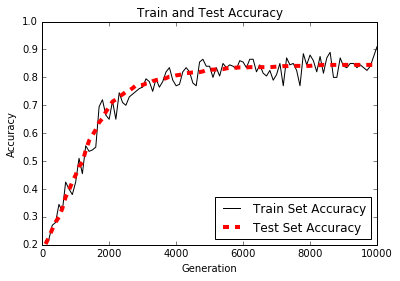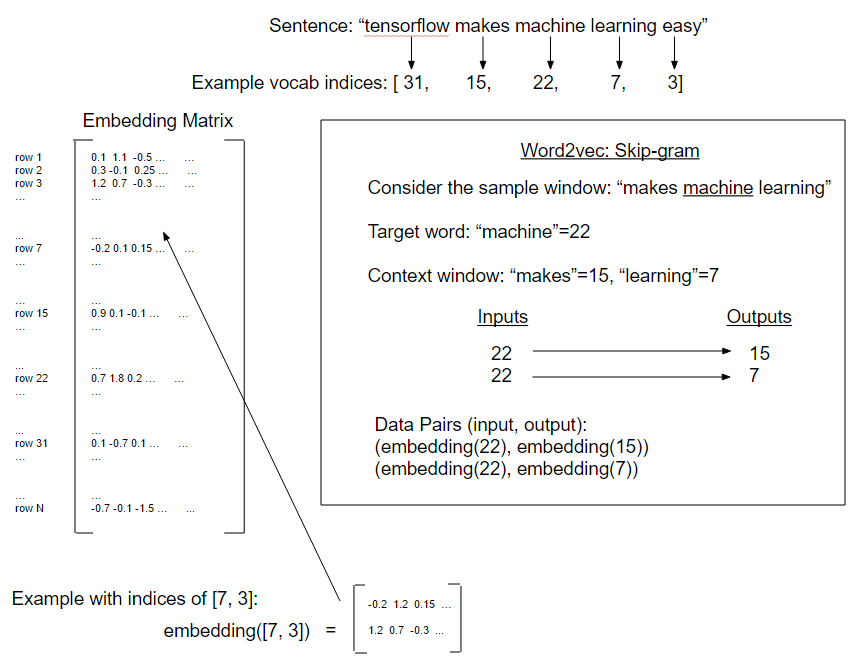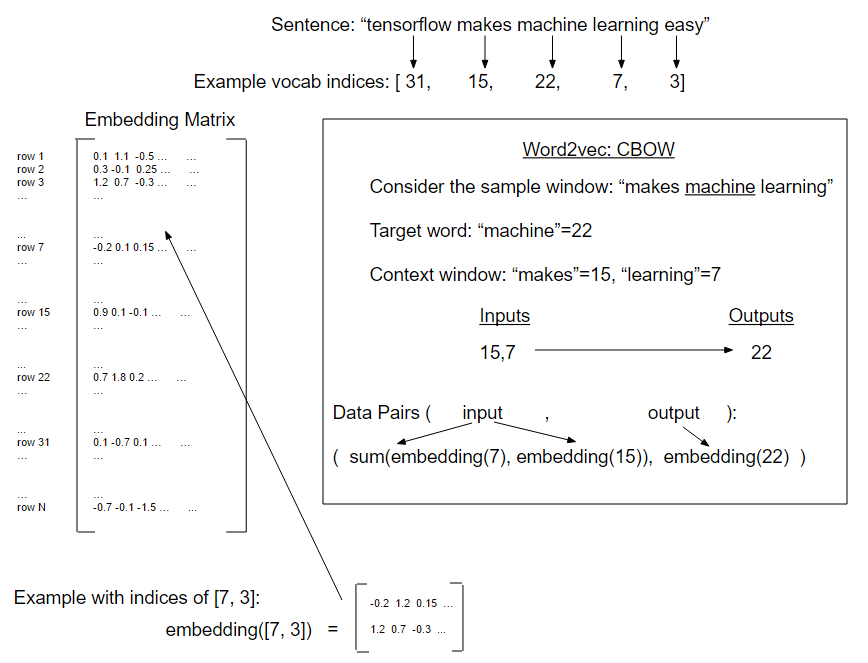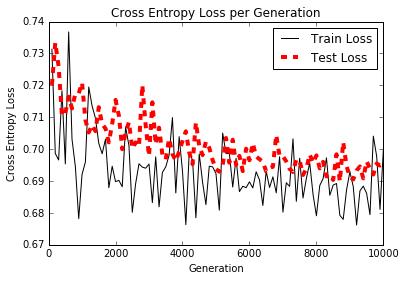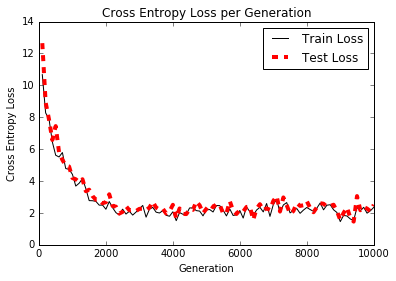Natural Language Processing (NLP) is a way of processing textual information into numerical summaries, features, or models. In this chapter we will motivate and explain how to best deal with text in TensorFlow. We show how to implement the classic 'Bag-of-Words' and show that there may be better ways to embed text based on the problem at hand. There are neural network embeddings called Word2Vec (CBOW and Skip-Gram) and Doc2Vec. We show how to implement all of these in TensorFlow.

• We introduce methods for turning text into numerical vectors. We introduce the TensorFlow 'embedding' feature as well.
• Here we use TensorFlow to do a one-hot-encoding of words called bag-of-words. We use this method and logistic regression to predict if a text message is spam or ham.
• We implement Text Frequency - Inverse Document Frequency (TFIDF) with a combination of Sci-kit Learn and TensorFlow. We perform logistic regression on TFIDF vectors to improve on our spam/ham text-message predictions.
• Our first implementation of Word2Vec called, "skip-gram" on a movie review database.
• Next, we implement a form of Word2Vec called, "CBOW" (Continuous Bag of Words) on a movie review database. We also introduce method to saving and loading word embeddings.
• In this example, we use the prior saved CBOW word embeddings to improve on our TF-IDF logistic regression of movie review sentiment.
• Here, we introduce a Doc2Vec method (concatenation of doc and word embeddings) to improve out logistic model of movie review sentiment.

## Ch 8: Convolutional Neural Networks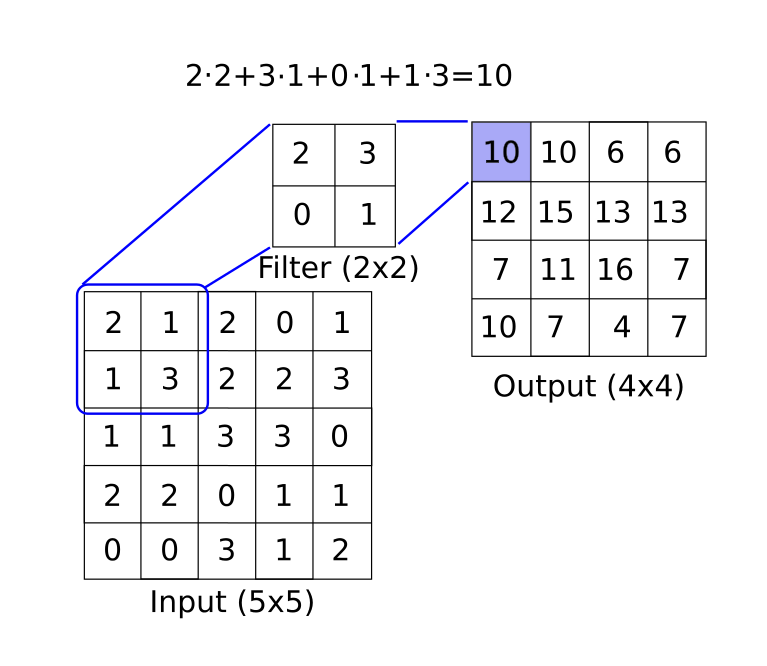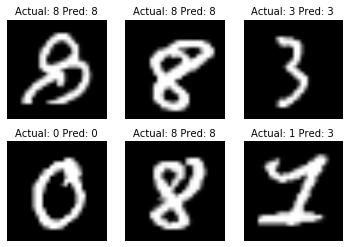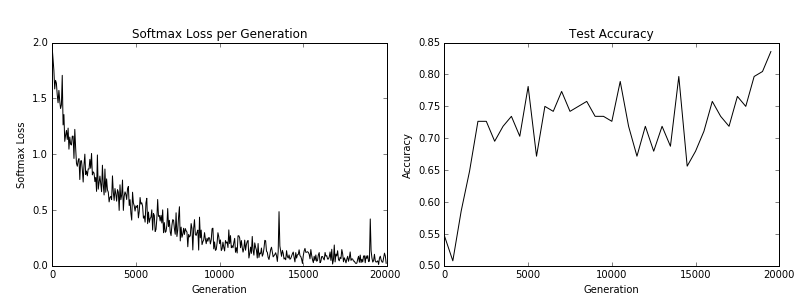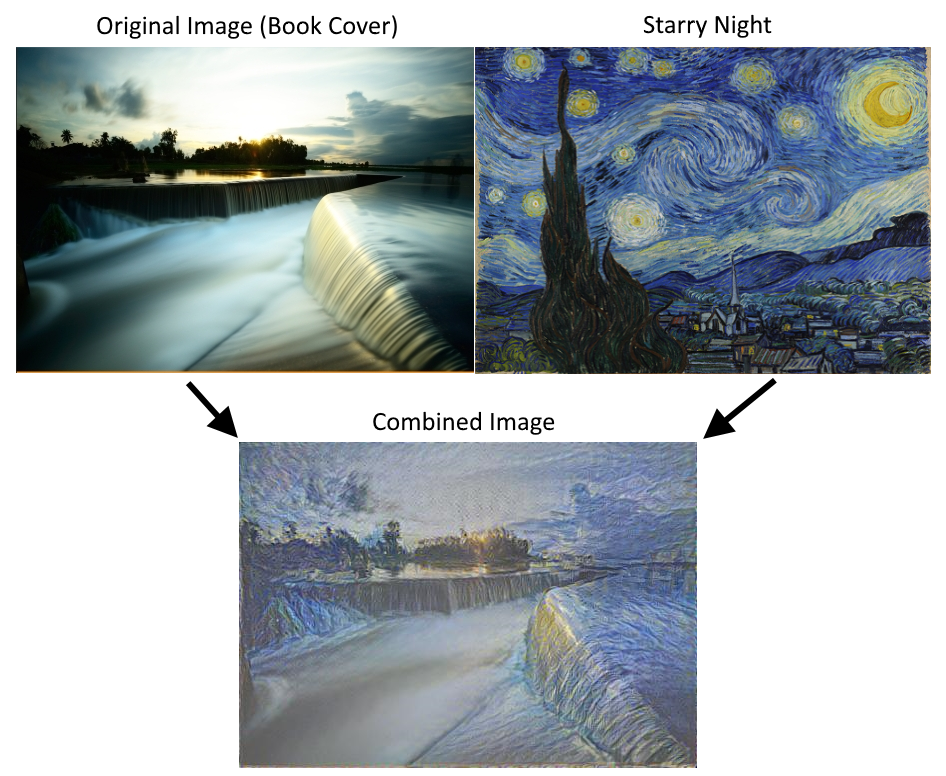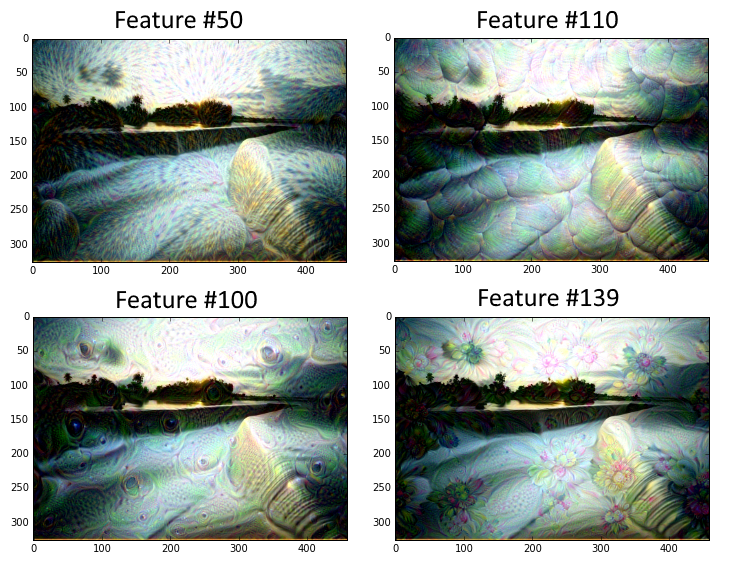Convolutional Neural Networks (CNNs) are ways of getting neural networks to deal with image data. CNN derive their name from the use of a convolutional layer that applies a fixed size filter across a larger image, recognizing a pattern in any part of the image. There are many other tools that they use (max-pooling, dropout, etc...) that we show how to implement with TensorFlow. We also show how to retrain an existing architecture and take CNNs further with Stylenet and Deep Dream.

• We introduce convolutional neural networks (CNN), and how we can use them in TensorFlow.
• Here, we show how to create a CNN architecture that performs well on the MNIST digit recognition task.
• In this example, we show how to replicate an architecture for the CIFAR-10 image recognition task.
• We show how to download and setup the CIFAR-10 data for the TensorFlow retraining/fine-tuning tutorial.
• In this recipe, we show a basic implementation of using Stylenet or Neuralstyle.
• This script shows a line-by-line explanation of TensorFlow's deepdream tutorial. Taken from Deepdream on TensorFlow. Note that the code here is converted to Python 3.

## Ch 9: Recurrent Neural Networks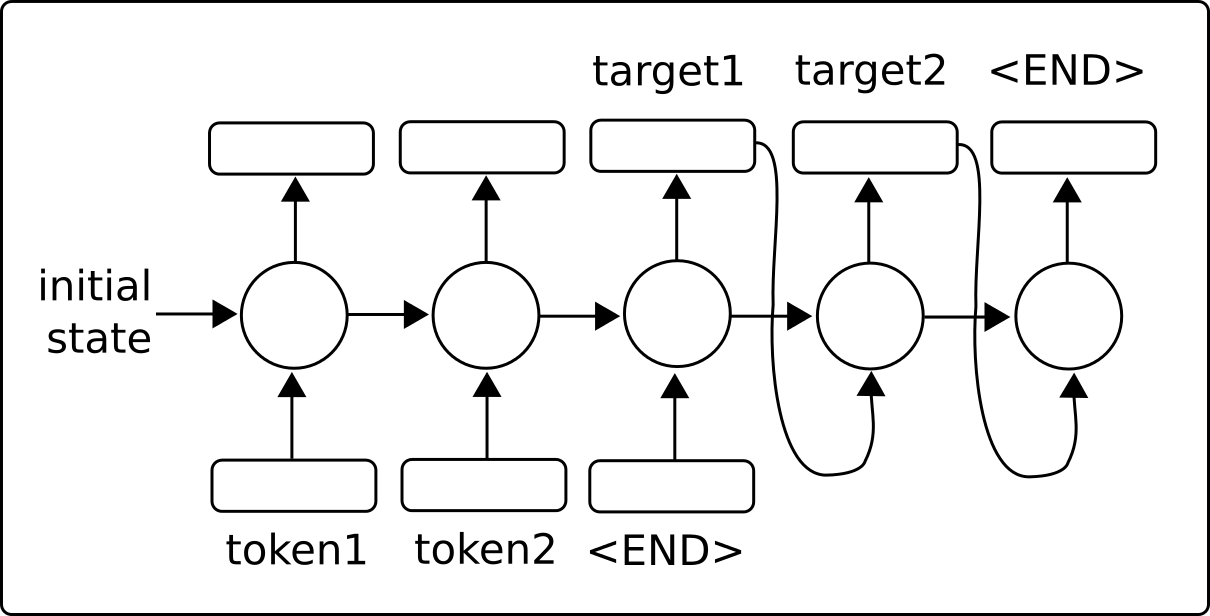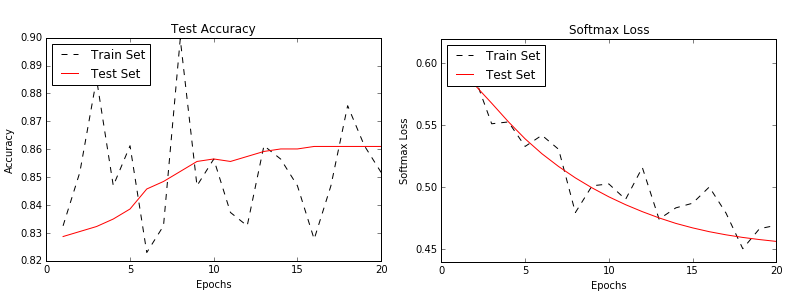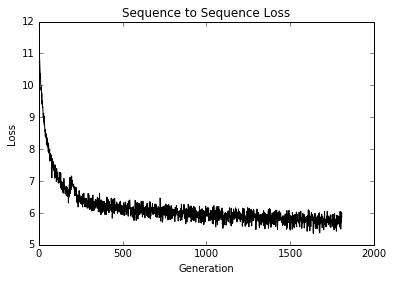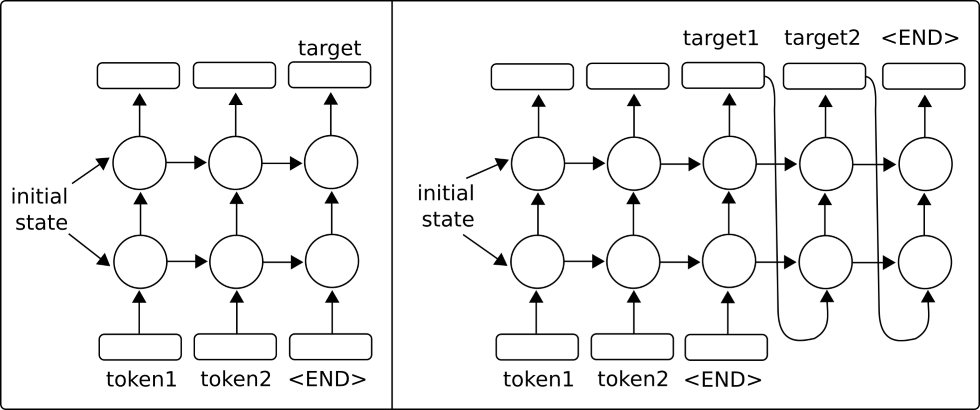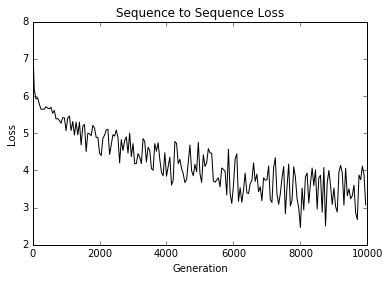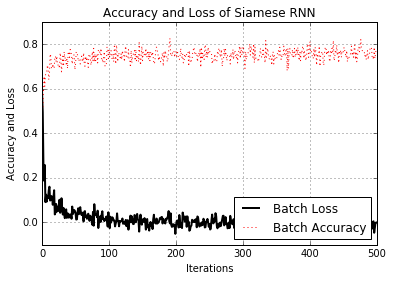Recurrent Neural Networks (RNNs) are very similar to regular neural networks except that they allow 'recurrent' connections, or loops that depend on the prior states of the network. This allows RNNs to efficiently deal with sequential data, whereas other types of networks cannot. We then motivate the usage of LSTM (Long Short Term Memory) networks as a way of addressing regular RNN problems. Then we show how easy it is to implement these RNN types in TensorFlow.

• We introduce Recurrent Neural Networks and how they are able to feed in a sequence and predict either a fixed target (categorical/numerical) or another sequence (sequence to sequence).
• In this example, we create an RNN model to improve on our spam/ham SMS text predictions.
• We show how to implement a LSTM (Long Short Term Memory) RNN for Shakespeare language generation. (Word level vocabulary)
• We stack multiple LSTM layers to improve on our Shakespeare language generation. (Character level vocabulary)
• Here, we use TensorFlow's sequence-to-sequence models to train an English-German translation model.
• Here, we implement a Siamese RNN to predict the similarity of addresses and use it for record matching. Using RNNs for record matching is very versatile, as we do not have a fixed set of target categories and can use the trained model to predict similarities across new addresses.

## Ch 10: Taking TensorFlow to ProductionOf course there is more to TensorFlow than just creating and fitting machine learning models. Once we have a model that we want to use, we have to move it towards production usage. This chapter will provide tips and examples of implementing unit tests, using multiple processors, using multiple machines (TensorFlow distributed), and finish with a full production example.

• We show how to implement different types of unit tests on tensors (placeholders and variables).
• How to use a machine with multiple devices. E.g., a machine with a CPU, and one or more GPUs.
• How to setup and use TensorFlow distributed on multiple machines.
• Various tips for developing with TensorFlow
• We show how to do take the RNN model for predicting ham/spam (from Chapter 9, recipe #2) and put it in two production level files: training and evaluation.

## Ch 11: More with TensorFlow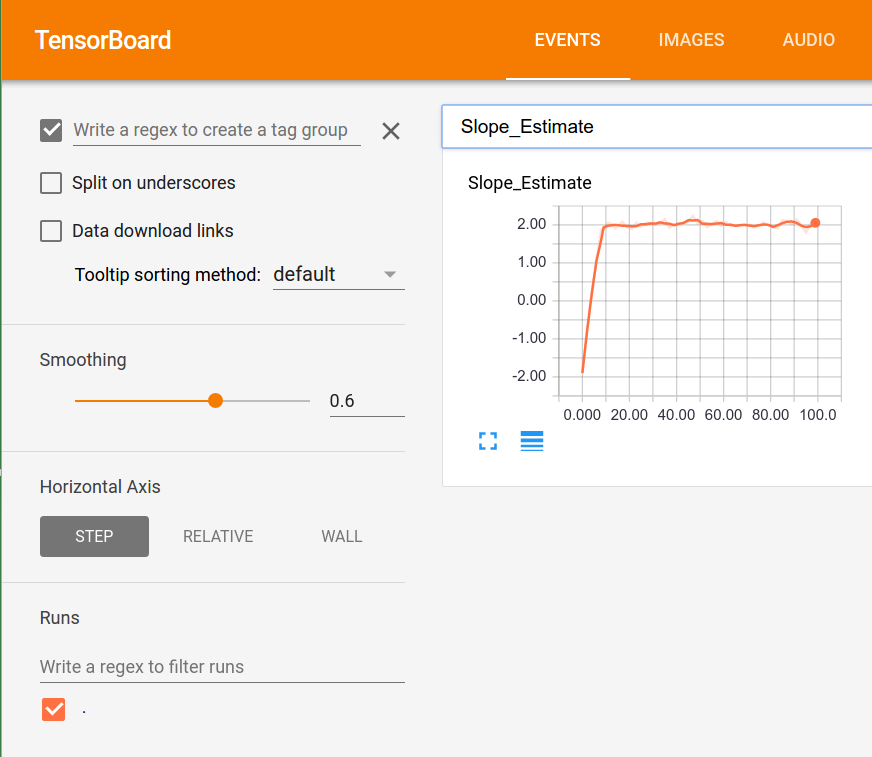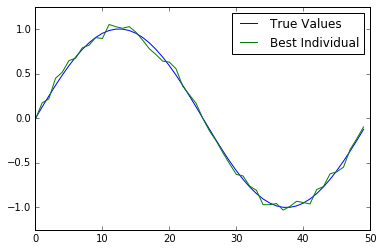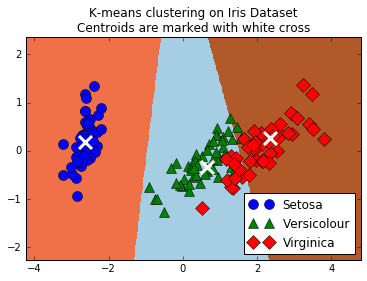To illustrate how versatile TensorFlow is, we will show additional examples in this chapter. We start with showing how to use the logging/visualizing tool Tensorboard. Then we illustrate how to do k-means clustering, use a genetic algorithm, and solve a system of ODEs.

• An example of using histograms, scalar summaries, and creating images in Tensorboard.
• We create a genetic algorithm to optimize an individual (array of 50 numbers) toward the ground truth function.
• How to use TensorFlow to do k-means clustering. We use the Iris data set, set k=3, and use k-means to make predictions.
• Here, we show how to use TensorFlow to solve a system of ODEs. The system of concern is the Lotka-Volterra predator-prey system.
• We illustrate how to use TensorFlow's gradient boosted regression and classification trees.
• Here we show how to use the Keras sequential model building for a fully connected neural network and a CNN model with callbacks.
Popular Tensorflow Projects
Popular Machine Learning Projects
Popular Machine Learning Categories
Related Searches

Get A Weekly Email With Trending Projects For These Categories
No Spam. Unsubscribe easily at any time.
Jupyter Notebook
Machine Learning
Tensorflow
Nlp
Neural Network
Classification
Cnn
Rnn
Svm
Genetic Algorithm
Linear Regression
Tensorboard
Packtpub